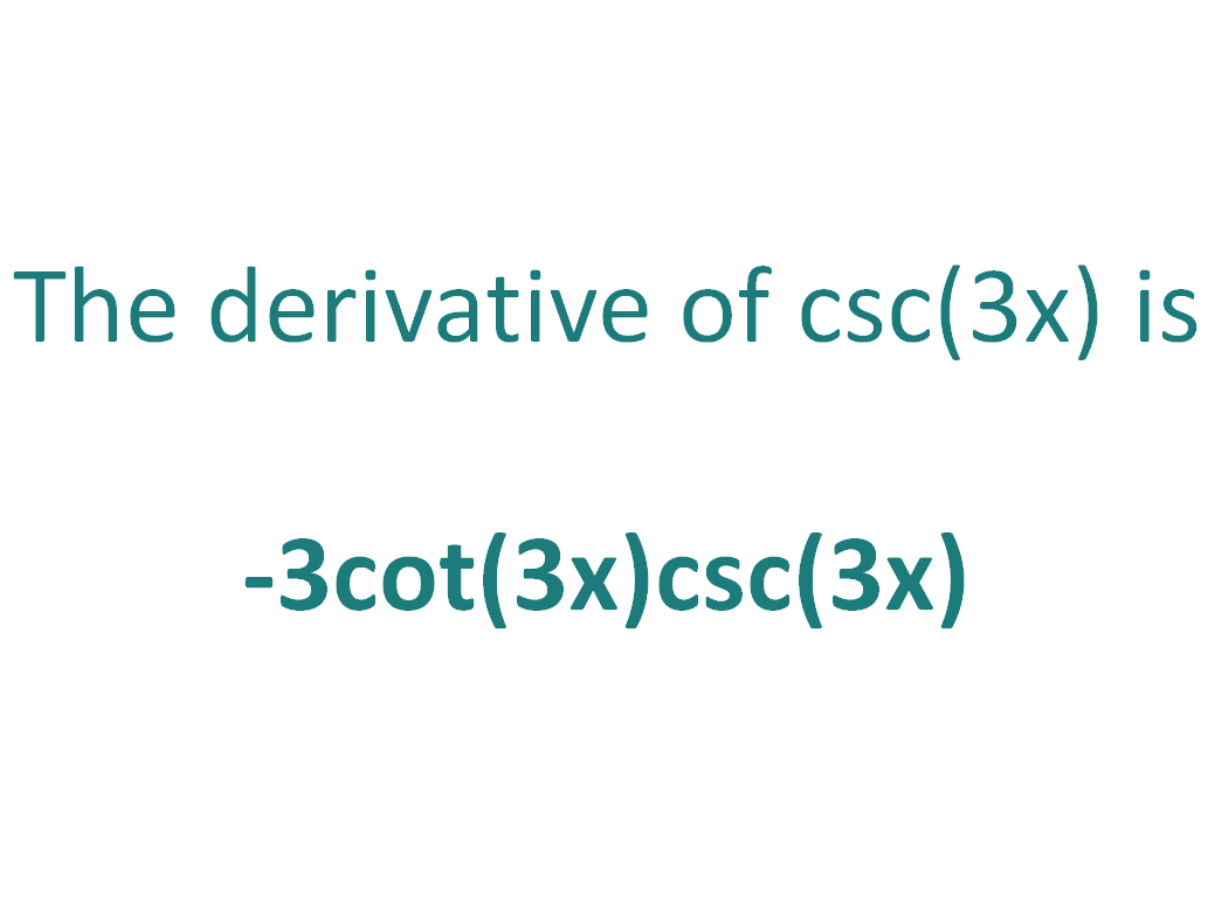# The Derivative of csc(3x)The derivative of csc(3x) is -3cot(3x)csc(3x)

## How to calculate the derivative of csc(3x)

The chain rule is useful for finding the derivative of a function which could have been differentiated had it been in x, but it is in the form of another expression which could also be differentiated if it stood on its own.

In this case:

• We know how to differentiate csc(x) (the answer is -csc(x)cot(x))
• We know how to differentiate 3x (the answer is 3)

This means the chain rule will allow us to perform the differentiation of the expression csc(3x).

#### Using the chain rule to find the derivative of csc(3x)

To perform the differentiation csc(3x), the chain rule says we must differentiate the expression as if it were just in terms of x as long as we then multiply that result by the derivative of what the expression is actually in terms of (in this case the derivative of 3x).

Let’s call the function in the argument of csc, g(x), which means the function is in the form of csc(x), except it does not have x as the angle, instead it has another function of x (3x) as the angle

If:

g(x) = 3x

It follows that:

csc(3x) = csc(g(x))

So if the function f(x) = cosec(x) and the function g(x) = 3x, then the function csc(3x) can be written as a composite function.

f(x) = csc(x)

f(g(x)) = csc(g(x)) (but g(x) = 3x)

f(g(x)) = csc(3x)

Let’s define this composite function as F(x):

F(x) = f(g(x)) = csc(3x)

We can find the derivative of csc(3x) (F'(x)) by making use of the chain rule.

The Chain Rule:
For two differentiable functions f(x) and g(x)

If F(x) = f(g(x))

Then the derivative of F(x) is F'(x) = f’(g(x)).g’(x)

Now we can just plug f(x) and g(x) into the chain rule.

How to find the derivative of csc(3x) using the Chain Rule:

Using the chain rule, the derivative of csc(3x) is -3cot(3x)csc(3x)

Finally, just a note on syntax and notation: csc(3x) is sometimes written in the forms below (with the derivative as per the calculations above). Just be aware that not all of the forms below are mathematically correct.

## The Second Derivative Of csc(3x)

To calculate the second derivative of a function, differentiate the first derivative.

From above, we found that the first derivative of csc(3x) = -3cot(3x)csc(3x). So to find the second derivative of csc(3x), we need to differentiate -3cot(3x)csc(3x).

We can use the product and chain rules, and then simplify to find the derivative of -3cot(3x)csc(3x) is 9csc3(3x) + 9cot2(3x)csc(3x)

The second derivative of csc(3x) is 9csc3(3x) + 9cot2(3x)csc(3x)